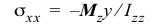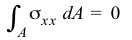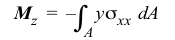# 6.1 Symmetric Bending of Beams

In the questions below, select the correct answer.

Name Email
1. If you know the bending normal strain at one point other than the centroid, then the bending normal strain can be found at any point on the cross section.
2. If you the maximum bending normal stress on a cross section, then the bending normal stress at any point on the cross section can be found for a linear-homogenous material.
3. A rectangular beam with a 2-in. × 4-in. cross section should be used with the 2-in. side parallel to the bending (transverse) forces.
4. The best place to drill a hole in a beam is through the centroid.
5. In the formulay is measured from the bottom of the beam.
6. The formulacan be used to find the normal stress on a cross section of a tapered beam.
7. The equationsandcannot be used for nonlinear materials.
8. The equationcan be used for nonhomogeneous cross sections.
9. The internal shear force jumps by the value of the applied transverse force as one crosses it from left to right.
10. The internal bending moment jumps by the value of the applied concentrated moment as one crosses it from left to right.